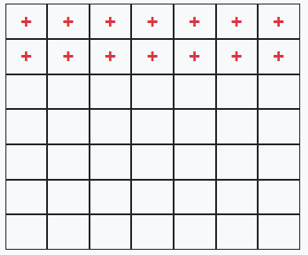Subtraction of fractions with different denominator

## Objective

To find the difference between two, unlike fractions.

## Pre-requisite Knowledge

• Concept of subtraction of natural numbers.
• Concept of fractions.
• Concept of grid method.

## What is Fraction?

A fraction is a numerical value that represents the parts of the whole.

## Parts of a Fraction

A fraction consists of two parts, namely the numerator and the denominator. The upper part of the fraction is called the numerator and the lower part of the fraction is called the denominator. For example, 4/7 is a fraction. Here, 4 is the numerator and 7 is the denominator.

## Unlike fraction

• Fractions with different denominators are called, unlike fractions.
• Thus, 1/5, 2/7, 3/2, and 8/6 are all unlike fractions.

### Grid representation of fraction7*7 grid

• In the above diagram, the total number of cells in the grid represents the denominator.
• The total number of cells coloured/marked represents the numerator.